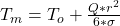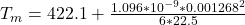## An electric current of 200 A is passed through a stainless steel wire having a radius R of 0.001268 m. The wire is L = 0.91 m long and has a

Question

An electric current of 200 A is passed through a stainless steel wire having a radius R of 0.001268 m. The wire is L = 0.91 m long and has a resistance R of 0.126 Ohm. The outer surface temperature Tw is held at 422.1 K. The average thermal conductivity is K = 22.5 W/mK. Calculate the center temperature. Power = I^2R (Watts) Where I = current in nmps and R = Resistance in ohms I^2R (Watts) = q pi(Radius)^2L

in progress 0
2 months 2021-07-26T18:12:28+00:00 1 Answers 13 views 0

The value is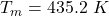Explanation:

From the question we are told that

The  current is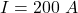The radius is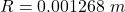The  length of the wire is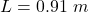\

The  resistance is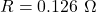The  outer surface temperature is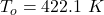The average thermal conductivity is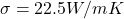Generally the heat generated in the stainless steel wire is mathematically represented as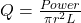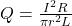=>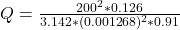=>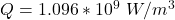Generally the middle temperature is mathematically represented as